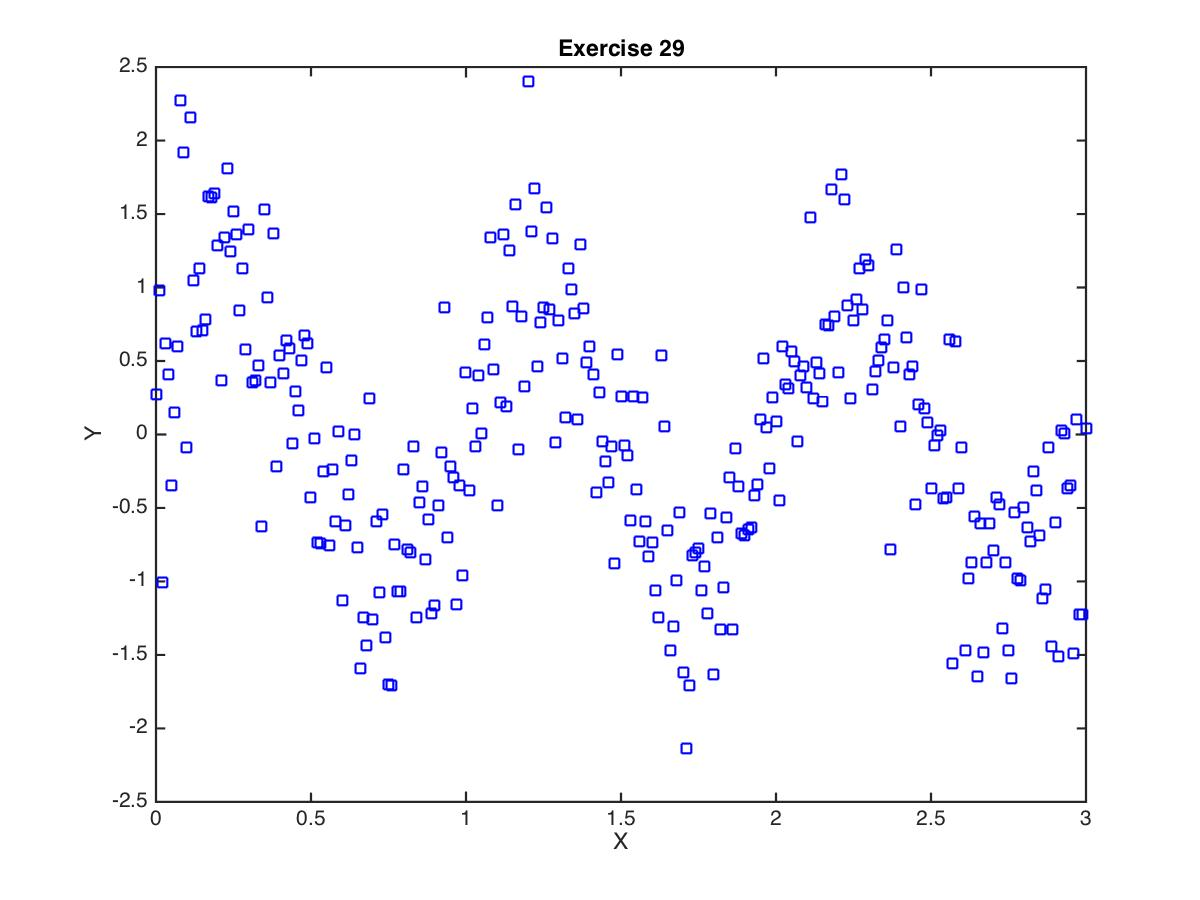# Exercise 29

## Local minima

Generate some (x,y) data by adapting the following MATLAB/Octave code to your language of choice:

x = 0:0.01:3;
y = sin(2*pi*x) + randn(size(x))*0.5;


Here's what the data look like (yours may look slightly different since the y values involve random values):Figure 1: (x,y) data for Exercise 29

Your task is to fit a function of the following form to the data:

\begin{equation} \hat{y} = \mathrm{sin}(\beta x) \end{equation}

The (single) parameter to be optimized is $$\beta$$. Your cost function $$J$$ is:

\begin{equation} J = \sum_{i=1}^{n} (\hat{y_{i}} - y_{i})^{2} \end{equation}

where $$n$$ is the number of $$(x,y)$$ pairs in the data.

Map the cost landscape

Compute the cost function $$J$$ for values of $$\beta$$ ranging from -10.0 to 10.0, and plot the cost landscape (plot $$J$$ as a function of $$\beta$$).

Optimize for beta

Use whatever optimization method you wish, to find the value of $$\beta$$ that minimizes the cost function $$J$$. Plot the data and plot the best fitting function. Justify that you have found the global minimum and not a local minimum.

Paul Gribble | fall 2014